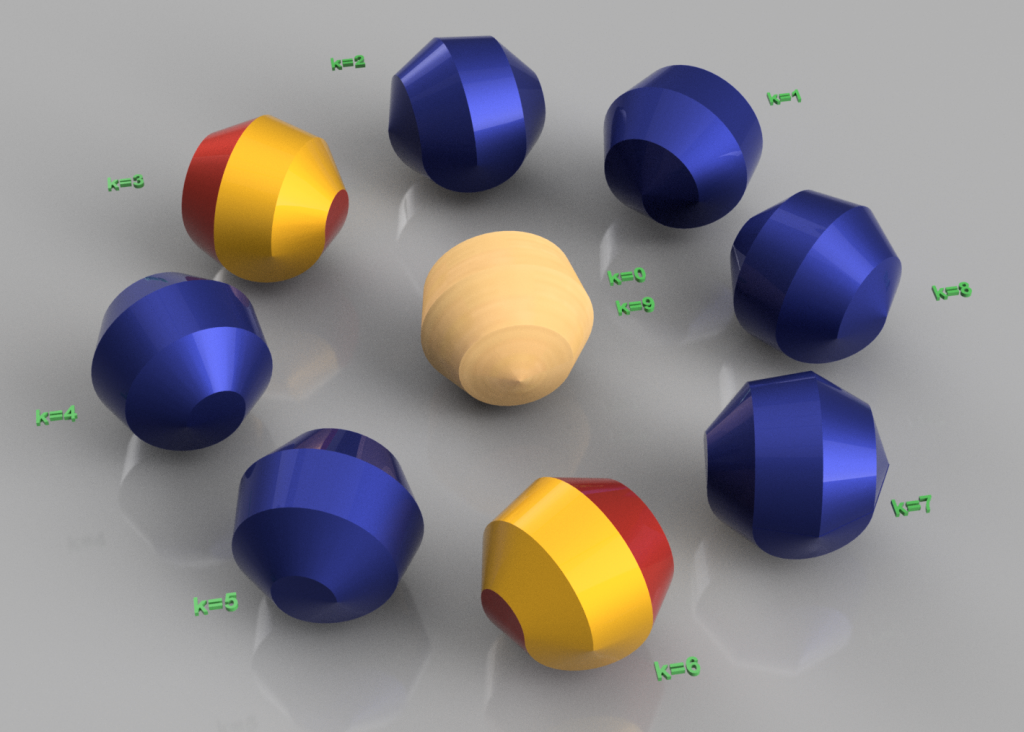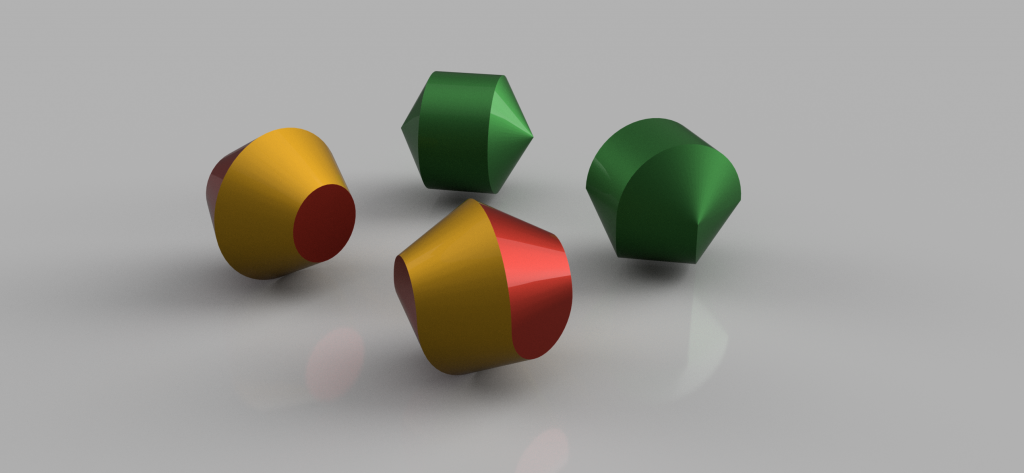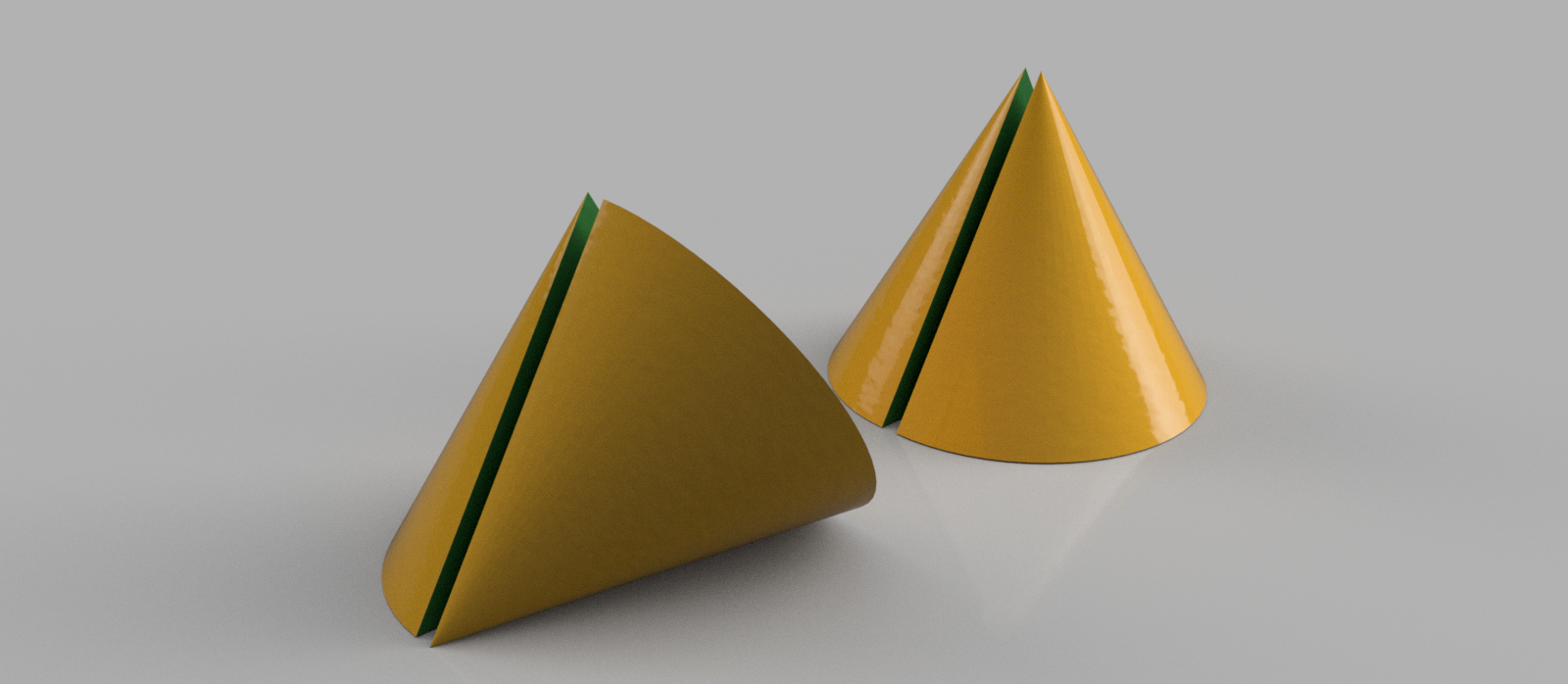# Polysphericons

1. September 2019

Polysphericons are solids which can be obtained from polygons with a revolution, cutting in half and gluing back together. One commonly refers to polysphericons as sphericons, we will stick to the correct names for clarity. Only the simple (4,1)-polysphericon (see below) also has the trivial name sphericon.

Depending on the polygon, different polysphericons can be generated. The common (4,1)-polysphericon (or just “sphericon”) can be obtained from the square. Depending on the chosen polygon different sphericons can be generated., e.g. the best-known sphericon is generated from a square:

The square is swept around the axis which passes through two corners and the center of mass, such that on obtains a bicone. This body of revolution is cut in half along the surface which passes the corners and center of the bicone. Lastly, the upper part is turned by 90 degrees along the vertical axis.

This sphericon appears similar to the oloid, but it is different in nature. You can find further information on the article about the oloid.

There are however many more sphericons to be generated in a similar manner: A polygon is revoluted to a body of revolution which is cut in half. These halves are then turned along the vertical axis. One of the more common sphericons is the hexasphericon made with the method above out of the hexagon.

Depending on the basic polygon (even /uneven number of corners) and the position of the rotational axis (corner-corner / corner-edge / edge-edge) on can assign to each solid a class of polysphericons:

• Dual polysphericon (even number of corners, rotational axis edge-edge)
• Even polysphericon (even number of corners, rotational axis corner-corner)
• Uneven polysphericon (uneven number of corners, rotational axis corner-edge)

With the number n of corners and the number k of distinct turns of the two halves one can read out the number of surfaces and edges of the polysphericon:

 Dual Polysphericon Even Polysphericon Uneven Polysphericon number of surfaces gcd(n/2,k) +1 gcd(n/2,k) (gcd(n,k) +1) /2 number of edges gcd(n/2,k) gcd(n/2,k) +1 (gcd(n,k) +1) /2

The abbreviation gcd stands for greatest common divisor.Uneven (9,k)-polysphericon with nonagon as underlying polygon. The value of k indicates how many steps the upper half has been turned. At values k=3 and k=6 the surface of the polysphericon consist of two separated surfaces. Obviously, the pattern repeats after nine turns. There is also another symmetry: A rotation with value k is equivalent to a rotation with (n-k) rotations. Equivalent in this context means that the solids are mirror-symmetric to each other. This is easy to see at the solids with k=4 and k=5, whose spirals are turning clockwise and anti-clockwise, respectively.In the upper right corner: body of revolution constructed from a hexagon with roational axis going through two corners. The other green solid is the hexasphericon, an even (6,1)-polysphericon. The yellow/red solid on the left is a body of revolution from a hexagon with rotational axis passing two edges of the hexagon. The corresponding dual (6,1)-polysphericon then consists of two separated surfaces which are indicated by different colors.

## Rolling Polysphericons

Not all polysphericons can roll. Only those polysphericons with gcd(n/2,k)=1, whose polygon has an even number of vertices and whose axis of revolution passes two corners can. These polysphericons are called “prime” polysphericons. Sphericon and hexasphericon are examples of rolling polysphericons.

However, looking at the (3,1)-polysphericon below on the left, one can easily understand that the rolling comes to an immediate stop when the polysphericon lies on one flat side. The same holds for dual polysphericons. They either possess several surfaces, such that stop abruptly or they roll in a circle like a spin top with too little energy.

Prime polysphericons have the property of a constant height of the center of mass. They thus roll relatively smoothly. The higher the order n of the solid, the less smoothly it rolls however. This is due to the fact, that the polysphericon needs to change the rolling direction more frequently, the more numerous the stripes of the surface are.On the left: (3,1)-polysphericon with gap to show its construction. On the right: Cone as body of revolution with axis passig one edge and one corner.